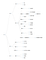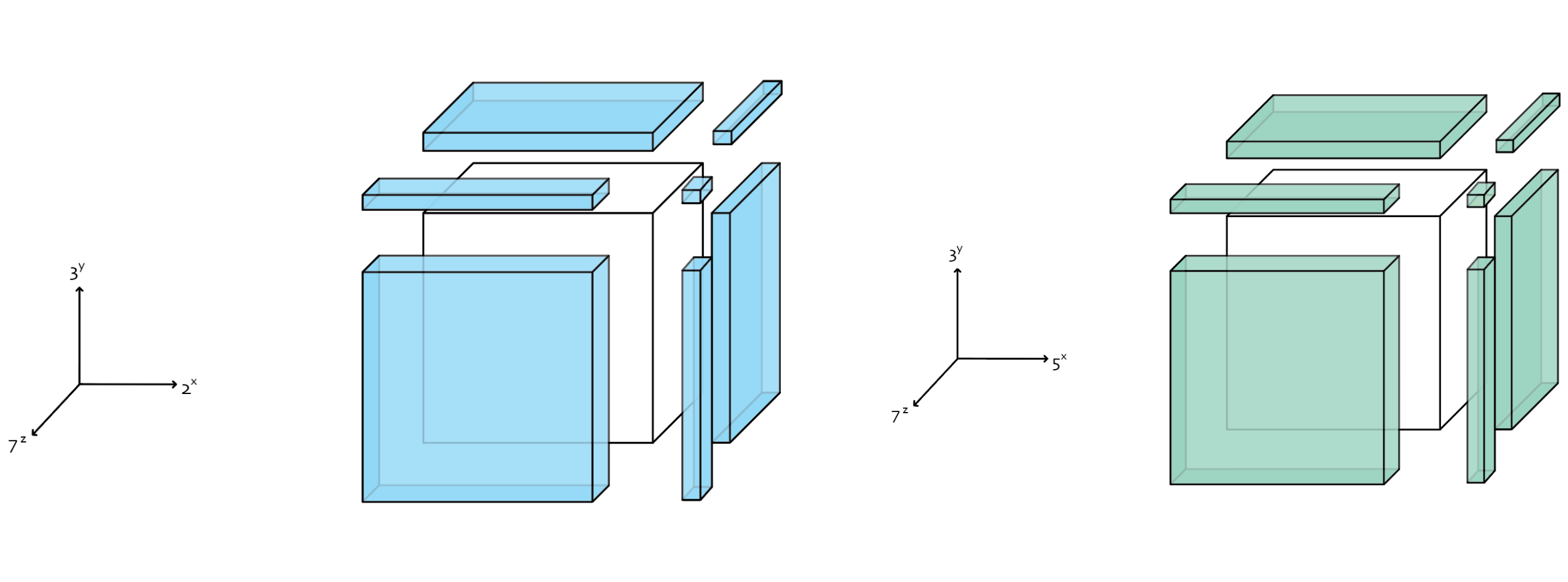# The Sequence

As with many sequences, multiplicative persistence (MP) is perhaps best explained by example, not jargon.

The MP of 35 is 1 because: 3 × 5 = 15, 1 × 5 = 5
The MP of 55 is 2 because: 5 × 5 = 25, 2 × 5 = 10, 1 × 0 = 0

See the pattern? Simply multiply a number’s digits together, and repeat the process until you arrive at a number between 0 and 9. A number’s MP is the number of rounds completed before the sequence terminates. Guessing random numbers, however, quickly shows that most sequences quickly terminate. Once a number contains a zero in any digit, it abruptly ends.

Naturally, mathematicians saw this pattern and challenged one another to find a number with the highest MP. So far, the longest sequence we’ve found has an MP of 11:

277777788888899 → 4996238671872 → 438939648 → 4478976 → 338688 → 27648 → 2688 → 768 → 336 → 54 → 20 → 0

If you’d like some more context, consider watching this video from Numberphile. Regardless, the record has held for decades and no one has been able to expand the series. By my calculations, it’s effectively impossible.

# The Algorithm

We’ve have been looking at MP in the wrong direction. We could naively count up and calculate MP(10) = 1, MP(11) = 1, MP(12) = 1… and so on. But if we take a ground-up approach, we can solve for all MP sequences in a fraction of the time.

The solutions can be found in all products of 2ˣ, 3ˣ, 5ˣ, and 7ˣ to build tree diagrams. Any non-trivial solution to an MP sequence must have two features:

• The number must be a product of 2ˣ, 3ˣ, 5ˣ, and 7ˣ.
• The number cannot contain a zero.Tree for all known sequences ending in 8. Note that each sequence can be extended by one node. The node “49” can be extended by a number containing any number created by two 7s and any number of 1s.

The number 23, for example is a “dead” branch. Its only factors are 1 and 23, so there’s no way that an upstream node can reach it. Additionally, we don’t care about tracking the number 20 because it quickly falls to zero. From now on I’ll consider any product that contains a zero as “trivial”, since any number can have an MP of 1. We are interested in longer chains.

On the other hand, we keep track of the number 36 because it doesn’t have a zero and it can be approached by a larger node. Any combination of {9, 4} or {3, 3, 2, 2}, {3, 3, 4} and so on (along with any number of ones) are viable upstream nodes—94, 194, 3143. And once any of those numbers become a product of any combination of 2ˣ, 3ˣ, 5ˣ, and 7ˣ, we can then add those numbers to our tree. The numbers 49 and 343, for example, are upstream nodes of 36 that can themselves be defined by the products 7² and 7³ respectively. 343 can be approached by 1777, 71177, 777, etc.

We can build an algorithm to search all possibilities of viable upstream nodes. The program iterates through every combination of 2ˣ, 3ˣ, 5ˣ, and 7ˣ, trying to find non-trivial products, writing them to a dictionary that could be saved (pickled) for later use. If the algorithm discovers a product without any zero digits, it is added to a dictionary that later can be used to construct the MP trees like the one above. The jupyter notebook can be found at this link.

An important optimization was separating the {2ˣ, 3ˣ, 7ˣ} search from the {3ˣ, 5ˣ, 7ˣ} search, since multiplying 2ˣ and 5ˣ would result in a factor of 10, inherently giving the number a zero in the ones place. This dropped search from O(N⁴) to O(N³).Visual diagram of how the algorithm expands the search space. The white cubes are the products we’ve already searched. We then expand the cube by segmenting our for-loops into the rectangular prisms shown in color. Allowing the algorithm to expand on an existing cube was important for multiprocessing.

Allowing the code to expand on a given search “cube” allowed for easy multiprocessing. In only a few days, I was able to cover all numbers up to 10⁵²⁵, or more than a googol googol googol googol googol. (It’s a large number.) However, there were no new additions to our MP tree after 10¹⁴⁰, which revealed the last number:

31262221321885653647626425815737111943458241183262682285162767533715254994149452428386848427865279586287233185834769954797571297422117699584

This number is 140 digits long, and is the largest known product of 2, 3, 5, or 7 that does not contain a zero. It can be written as 2²⁵ ∙ 3²²⁷ ∙ 7²⁸. Despite its huge length, however, it has an MP of 2. (And its infinite number of parent nodes—combinations of 1’s with the appropriate number of 2’s, 3’s, and 7’s—has an MP of 3.) Because this number contains 2’s and 5’s, the next number in the sequence contains a factor of 10, which immediately reduces the sequence to zero.

All known non-trivial solutions to 2ˣ, 3ˣ, 5ˣ, and 7ˣ can be found at this txt file.

It turns out that I wasn’t the first to think of this approach. In 2016, an IBM researcher and sequence enthusiast Benjamin Chaffin had made a similar discovery.

Numberphile incorrectly reported that the highest number up tested was 10²³³. They likely were drawing from an outdated Wikipedia and Wolfram Alpha page. Chaffin published his findings on the OEIS page, which I should have probably checked before jumping into the project. The Wikipedia page has been updated to reflect the expanded limit; however, the Wolfram Alpha page has not.

According to Chaffin, he ran the program for months, reached a new limit of 20,000 digits (a very *very* large number). He claims that the chain of length 11 still holds.

Questions? Thoughts? Contact me and see more projects on my website.

🏳️‍🌈 Machine learning engineer and data journalist. Learn about me and my projects at www.BrownAnalytics.com

## More from Kevin McElwee

🏳️‍🌈 Machine learning engineer and data journalist. Learn about me and my projects at www.BrownAnalytics.com